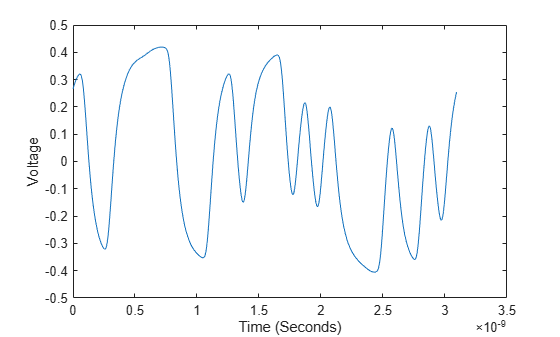Main Content

# pulse2wave

Data pattern waveform from pulse response

## Syntax

``W = pulse2wave(P,D,N)``

## Description

example

````W = pulse2wave(P,D,N)` converts the pulse response `P` into a voltage waveform `W`, given the symbol pattern `D` and the number of samples per symbol `N`.The function uses a circular convolution technique to project the pulse response onto the data pattern.```

## Examples

collapse all

Load the pulse response column matrix from a file.

`load('PulseResponseReflective100ps.mat');`

Find the pulse response column vector.

`P = pulse(:,1) - pulse(1,1);`

Create the symbol pattern of PRBS order 5.

`data = prbs(5,2^5-1)-0.5;`

Calculate and plot the voltage waveform from the pulse response.

```waveform = pulse2wave(P,data,SamplesPerSymbol); t = dt*(0:length(waveform)-1); figure plot(t,waveform) xlabel('Time (Seconds)') ylabel('Voltage')```## Input Arguments

collapse all

Input pulse response, specified as a column matrix. The first column contains the primary pulse response and the subsequent columns (if any) contain the crosstalk pulse responses.

Data Types: `double`

Symbol pattern, specified as a vector.

Data Types: `double`

Number of samples per symbol, specified as a positive integer scalar.

Data Types: `double`

## Output Arguments

collapse all

Voltage waveform data pattern, returned as a column matrix.

Data Types: `double`

## See Also

Introduced in R2020a

## SupportGet trial now